# PSAT Math : How to find the diameter of a sphere

## Example Questions

### Example Question #191 : Psat Mathematics

If a sphere has an approximate volume of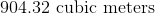, then what is the approximate diameter of this sphere?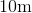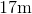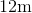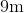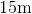Explanation:

The formula for the volume of a sphere is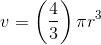Therefore,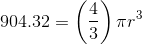Dividing both sides by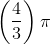, leaves us with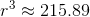. Taking the cube root, we find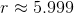, meaning our diameter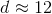.